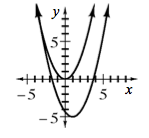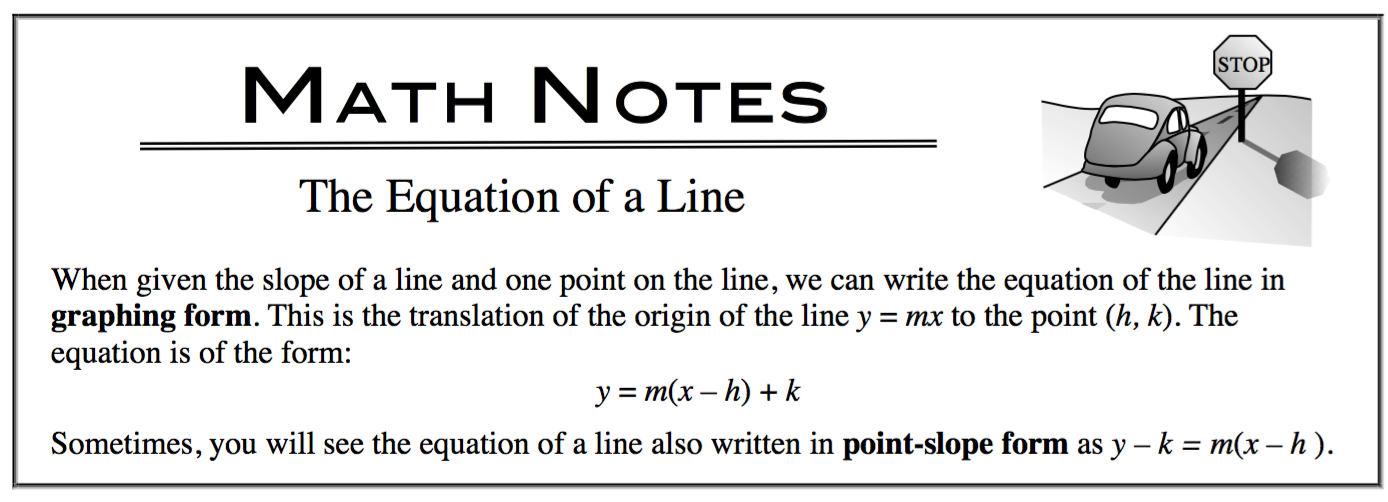### Home > APCALC > Chapter 1 > Lesson 1.1.1 > Problem1-7

1-7.

TRANSLATING FUNCTIONS Homework Help ✎1. Graph the function $y=\frac{2}{3}x^2$. On the same set of axes graph a translation of the function that is shifted $1$ unit to the right and $5$ units down. Write the equation of the translated function.

$y=\frac{2}{3}(x-1)^2-5$2. Does the same strategy work for $y =\frac { 2 } { 3 }x$ ? Write an equation that will shift $y =\frac { 2 } { 3 }x$ , $1$ unit to the right and $5$ units down.

$y=\frac{2}{3}(x-k)+v\ \ \ \ \ \ \ \ \text{What are }k\text{ and } v?$

3. Compare the graphs of $y = -\frac { 1 } { 2 }x$ and $y = -\frac { 1 } { 2 }(x + 2) + 3$. Describe their similarities and differences.

Both graphs are straight lines with the same slope. The second graph has been shifted both horizontally and vertically from

$y = -\frac{1}{2}x.$

4. Explain how you know that the graph of $y = -9(x + 1) - 6$ goes through the point $(-1, -6)$ and has a slope of $–9$.

This is a straight line in Point-Slope form. See Math Note for more information.5. Sketch the graph of $y = 5(x - 2) - 1$.

Refer to the hint in part (d).